## Polynomial

A Polynomial is a mathematical expression involving a series of Powers in one or more variables multiplied by Coefficients. A Polynomial in one variable with constant Coefficients is given by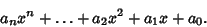(1)

The highest Power in a one-variable Polynomial is called its Order. A Polynomial in two variables with constant Coefficients is given by(2)

Exchanging the Coefficients of a one-variable Polynomial end-to-end produces a Polynomial(3)

whose Roots are Reciprocals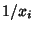of the original Roots.

The following table gives special names given to polynomials of low orders.

 Order Polynomial Type 1 Linear Equation 2 Quadratic Equation 3 Cubic Equation 4 Quartic Equation 5 Quintic Equation 6 Sextic Equation

Polynomials of fourth degree may be computed using three multiplications and five additions if a few quantities are calculated first (Press et al. 1989):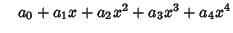(4)
where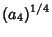(5)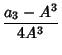(6)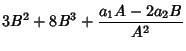(7)(8)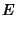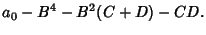(9)

Similarly, a Polynomial of fifth degree may be computed with four multiplications and five additions, and a Polynomial of sixth degree may be computed with four multiplications and seven additions.

Polynomials of orders one to four are solvable using only rational operations and finite root extractions. A first-order equation is trivially solvable. A second-order equation is soluble using the Quadratic Equation. A third-order equation is solvable using the Cubic Equation. A fourth-order equation is solvable using the Quartic Equation. It was proved by Abel(and Galois) using Group Theory that general equations of fifth and higher order cannot be solved rationally with finite root extractions.

However, the general Quintic Equation may be given in terms of the Theta Functions, or Hypergeometric Functions in one variable. Hermiteand Kroneckerproved that higher order Polynomials are not soluble in the same manner. Klein showed that the work of Hermite was implicit in the Group properties of the Icosahedron. Klein's method of solving the quintic in terms of Hypergeometric Functions in one variable can be extended to the sextic, but for higher order Polynomials, either Hypergeometric Functions in several variables or Siegel functions'' must be used. In the 1880s, Poincarécreated functions which give the solution to theth order Polynomial equation in finite form. These functions turned out to be natural'' generalizations of the Elliptic Functions.

Given anth degree Polynomial, the Roots can be found by finding the Eigenvalues of the Matrix(10)

This method can be computationally expensive, but is fairly robust at finding close and multiple roots.

Polynomial identities involving sums and differences of like Powers include(11)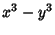(12)(13)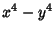(14)(15)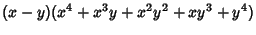(16)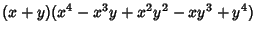(17)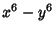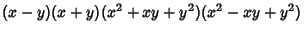(18)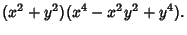(19)

Further identities include(20)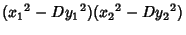(21)(22)

The identity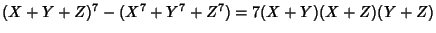(23)
was used by Lamé in his proof that Fermat's Last Theorem was true for.

See also Appell Polynomial, Bernstein Polynomial, Bessel Polynomial, Bezout's Theorem, Binomial, Bombieri Inner Product, Bombieri Norm, Chebyshev Polynomial of the First Kind, Chebyshev Polynomial of the Second Kind, Christoffel-Darboux Formula, Christoffel Number, Complex Number, Cyclotomic Polynomial, Descartes' Sign Rule, Discriminant (Polynomial), Durfee Polynomial, Ehrhart Polynomial, Euler Four-Square Identity, Fibonacci Identity, Fundamental Theorem of Algebra, Fundamental Theorem of Symmetric Functions, Gauss-Jacobi Mechanical Quadrature, Gegenbauer Polynomial, Gram-Schmidt Orthonormalization, Greatest Lower Bound, Hermite Polynomial, Hilbert Polynomial, Irreducible Polynomial, Isobaric Polynomial, Isograph, Jensen Polynomial, Kernel Polynomial, Krawtchouk Polynomial, Laguerre Polynomial, Least Upper Bound, Legendre Polynomial, Liouville Polynomial Identity, Lommel Polynomial, Lukács Theorem, Monomial, Orthogonal Polynomials, Perimeter Polynomial, Poisson-Charlier Polynomial, Pollaczek Polynomial, Polynomial Bar Norm, Quarter Squares Rule, Ramanujan 6-10-8 Identity, Root, Runge-Walsh Theorem, Schläfli Polynomial, Separation Theorem, Stieltjes-Wigert Polynomial, Trinomial, Trinomial Identity, Weierstraß's Polynomial Theorem, Zernike Polynomial

References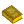Polynomials

Barbeau, E. J. Polynomials. New York: Springer-Verlag, 1989.

Bini, D. and Pan, V. Y. Polynomial and Matrix Computations, Vol. 1: Fundamental Algorithms. Boston, MA: Birkhäuser, 1994.

Borwein, P. and Erdélyi, T. Polynomials and Polynomial Inequalities. New York: Springer-Verlag, 1995.

Cockle, J. Notes on the Higher Algebra.'' Quart. J. Pure Applied Math. 4, 49-57, 1861.

Cockle, J. Notes on the Higher Algebra (Continued).'' Quart. J. Pure Applied Math. 5, 1-17, 1862.

Press, W. H.; Flannery, B. P.; Teukolsky, S. A.; and Vetterling, W. T. Numerical Recipes in C: The Art of Scientific Computing. Cambridge, England: Cambridge University Press, 1989.

Project Mathematics! Polynomials. Videotape (27 minutes). California Institute of Technology. Available from the Math. Assoc. Amer.